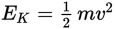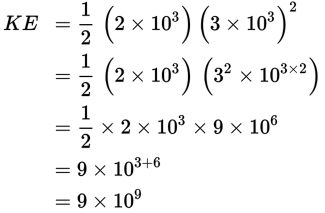# SAT Math Multiple Choice Question 348: Answer and Explanation

### Test Information

Question: 348

3. Kinetic energy is the energy of motion. The equationrepresents the kinetic energy in joules of an object with a mass of m kg traveling at a speed of v meters per second. What is the kinetic energy in joules of an unmanned aircraft with a mass of 2 × 103 kg traveling at a speed of approximately 3 × 103 meters per second?

• A. 9 × 59
• B. 9 × 108
• C. 9 × 109
• D. 1.8 × 1010

Explanation:

C

Difficulty: Easy

Category: Passport to Advanced Math / Exponents

Strategic Advice: You do not need a calculator to answer this question-just use the rules of exponents. Remember, when raising a power to a power, you multiply the exponents.

Getting to the Answer: Substitute the values given in the question into the formula. Then simplify using the rules of exponents.